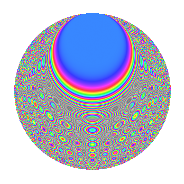# Properties

 Label 2100.2.nLevel 2100 Weight 2 Character orbit n Rep. character $$\chi_{2100}(1751,\cdot)$$ Character field $$\Q$$ Dimension 228 Sturm bound 960

# Related objects

## Defining parameters

 Level: $$N$$ $$=$$ $$2100 = 2^{2} \cdot 3 \cdot 5^{2} \cdot 7$$ Weight: $$k$$ $$=$$ $$2$$ Character orbit: $$[\chi]$$ $$=$$ 2100.n (of order $$2$$ and degree $$1$$) Character conductor: $$\operatorname{cond}(\chi)$$ $$=$$ $$12$$ Character field: $$\Q$$ Sturm bound: $$960$$

## Dimensions

The following table gives the dimensions of various subspaces of $$M_{2}(2100, [\chi])$$.

Total New Old
Modular forms 504 228 276
Cusp forms 456 228 228
Eisenstein series 48 0 48

## Trace form

 $$228q + 4q^{4} - 6q^{6} - 4q^{9} + O(q^{10})$$ $$228q + 4q^{4} - 6q^{6} - 4q^{9} - 6q^{12} - 20q^{16} + 20q^{18} - 2q^{24} + 8q^{28} - 16q^{33} - 32q^{34} + 32q^{36} + 16q^{37} - 10q^{42} + 48q^{46} - 34q^{48} - 228q^{49} - 12q^{52} - 10q^{54} + 32q^{57} - 56q^{58} + 16q^{61} + 52q^{64} - 40q^{66} + 24q^{69} + 8q^{72} + 24q^{73} - 12q^{76} + 72q^{78} + 12q^{81} + 24q^{82} - 14q^{84} + 80q^{88} + 72q^{93} + 152q^{94} + 74q^{96} - 24q^{97} + O(q^{100})$$

## Decomposition of $$S_{2}^{\mathrm{new}}(2100, [\chi])$$ into newform subspaces

The newforms in this space have not yet been added to the LMFDB.

## Decomposition of $$S_{2}^{\mathrm{old}}(2100, [\chi])$$ into lower level spaces

$$S_{2}^{\mathrm{old}}(2100, [\chi]) \cong$$ $$S_{2}^{\mathrm{new}}(60, [\chi])$$$$^{\oplus 4}$$$$\oplus$$$$S_{2}^{\mathrm{new}}(84, [\chi])$$$$^{\oplus 3}$$$$\oplus$$$$S_{2}^{\mathrm{new}}(300, [\chi])$$$$^{\oplus 2}$$$$\oplus$$$$S_{2}^{\mathrm{new}}(420, [\chi])$$$$^{\oplus 2}$$

## Hecke characteristic polynomials

There are no characteristic polynomials of Hecke operators in the database